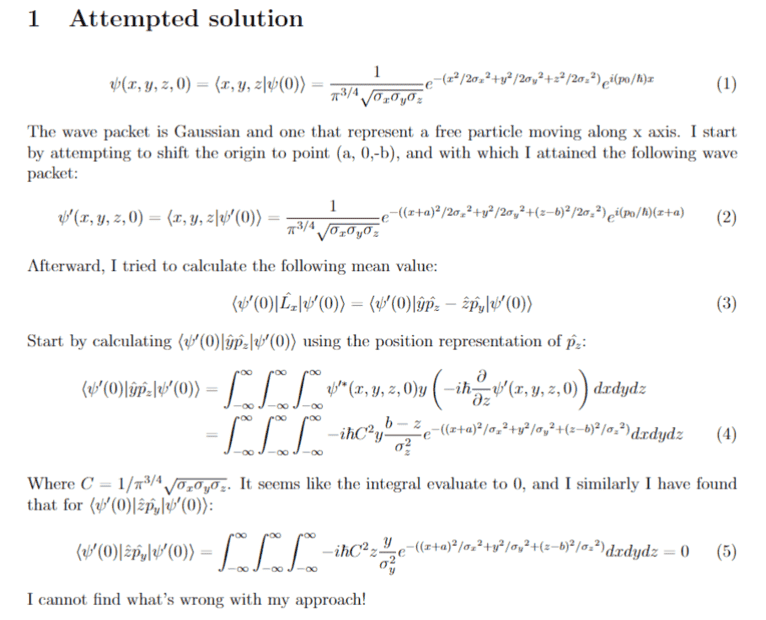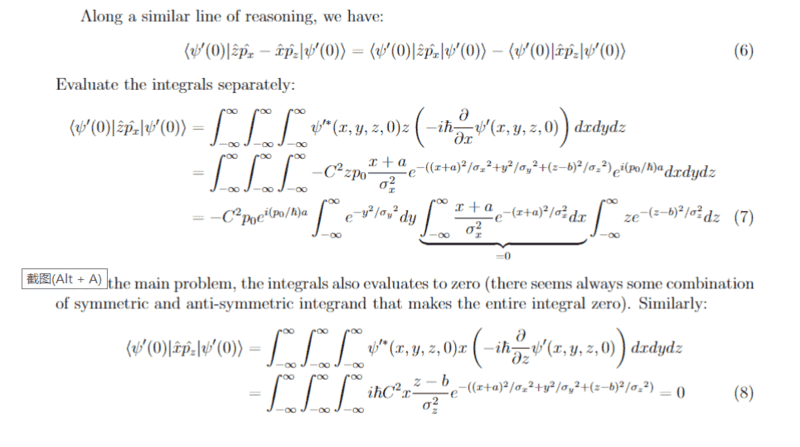# Average value of components of angular momentum for a wave packet

• Nelsonc
In summary, the conversation discusses a problem with integrals evaluating to 0 in classical mechanics involving a free particle with nonzero angular momentum with respect to the y axis. The individual has already typed up the problem in latex and has shown their work for the y and z components of angular momentum, but still gets a result of 0. They mention a potential issue with the method and the antisymmetric nature of the integrand. Another person points out an error in the derivation of equation (7) and the individual realizes their mistake, leading to a result of ##bp_0##.

#### Nelsonc

Homework Statement
Given a wave packet as shown (see below), find the mean value of angular momentum components L_x, L_y, L_z with regard to point (a,0,-b) where a and b (the impact parameter) are nonzero
Relevant Equations
\frac{1}{\pi^{3/4} \sqrt{\sigma_x\sigma_y\sigma_z}}e^{-(x^2/2{\sigma_x}^2+y^2/2{\sigma_y}^2+z^2/2{\sigma_z}^2)}e^{i(p_0/\hbar)x}
I have typed up the main problem in latex (see photo below)It seems all such integrals evaluates to 0, but that is apparantly unreasonable for in classical mechanics such a free particle is with nonzero angular momentum with respect to y axis.

Nelsonc said:
It seems all such integrals evaluates to 0, but that is apparantly unreasonable for in classical mechanics such a free particle is with nonzero angular momentum with respect to y axis.
You didn't show your work for the y and z components of angular momentum. You shouldn't get zero for the y component.

Thanks for the reminder, but I have already done so and it turns out they all goes to 0, so there must be something awry with my method. In particular, there seems always to be one spacial part of the integrand to be antisymmetric so that the whole integral goes to 0 (please refer to the image attached). Moreover, I know that classically a free particle moving in such fashion would have y angular momentum component being ##bp_0##In deriving equation (7), check your result for ##\large \frac{\partial \psi'}{\partial x}##. Did you use the product rule when taking the derivative of the product of the Gaussian function and the function ##e^{i(p_0/\hbar)x}##?

I see, thanks so much for catching that error! Now the calculation generates ##bp_0## as a result.

•TSny